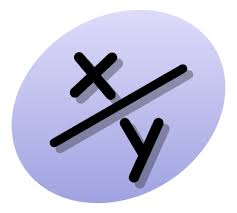# $x$, $y$, and $x$-ponents

Algebra Level 1If $\frac{4^{x}}{2^{x + y}}$ $= 8$ and $\frac{9^{x + y}}{3^{5y}}$ $= 243$, where $x$ and $y$ are real numbers, then what is the sum $x + y$?

×